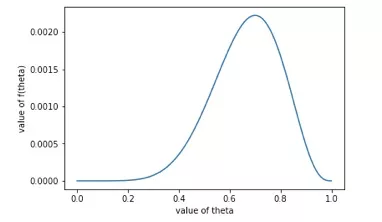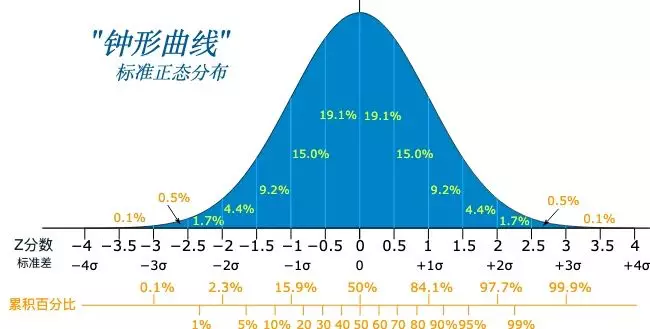# 机器学习基础——让你一文学会朴素贝叶斯模型

$P(A|B)=\frac{P(A)P(B|A)}{P(B)}$

### 似然函数

$P(x|\theta)=\theta^7*(1-\theta)^3=f(\theta)$

import numpy as np
import matplotlib.pyplot as plt

x = np.linspace(0, 1, 100)
y = np.power(x, 7) * np.power(1 - x, 3)

plt.plot(x, y)
plt.xlabel('value of theta')
plt.ylabel('value of f(theta)')
plt.show()### 模型详解

$P(A|B)=\frac{P(A)P(B|A)}{P(B)}$

$P(B)=\sum_{i=1}^mP(B|C_i)P(C_i)$

$P(y_i|x)=\frac{P(x|y_i)P(y_i)}{P(x)}$

$P(y_i|x)=P(y_i)P(a_1|y_i)P(a_2|y_i)\cdots P(a_n|y_i)=P(y_i)\prod_{j=1}^nP(a_j|y_i)$

$P(a_j|y_i)=\frac{N}{M}$

$P(a_j|y_i)=\frac{N+\alpha}{M+\beta}$$P(a_j|y_i)$是一个浮点数，而且很有可能非常小，而我们需要计算n个浮点数的乘积。由于存在精度误差，所以当连乘的结果小于精度的时候，就无法比较两个概率之间的大小了。

KaTeX parse error: No such environment: eqnarray at position 8: \begin{̲e̲q̲n̲a̲r̲r̲a̲y̲}̲ P(y_i|x) &= P(…©️2019 CSDN 皮肤主题: 数字20 设计师: CSDN官方博客